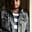Related Tags

python

# How to count the number of occurrences of a list item in PythonKedar Kodgire

## Introduction

Python provides us with different ways to count occurrences of list items. While certain ways are faster than others, this shot will provide you with a variety of methods that can be used.

As an exercise, try out all the methods in this shot to find the best method for yourself. Let me know in the comments which one you found to be the fastest!

Note: You can find the execution time of the code in the output once you run the code.

## 1. Count

count is the easiest to use of all methods. This method expects one argument, i.e., the list element whose number of occurrences are counted.

### Syntax

list.count(list_element)

Let’s look at an example.

l = ['a', 'a', 'a', 'a', 'a', 'b', 'b', 'b', 'b', 'c', 'c', 'c', 'd', 'd', 'e']
print(l.count('a'))
print(l.count('e'))


We can also use this method to get a count of all distinct values at once. For this purpose, we can use list comprehensions.

Comprehensions in Python provide us with a compact and concise way to build new sequences (lists, sets, dictionaries, etc.)

Let’s check out some examples.

l = ['a', 'a', 'a', 'a', 'a', 'b', 'b', 'b', 'b', 'c', 'c', 'c', 'd', 'd', 'e']

print([[x,l.count(x)] for x in set(l)])

print(dict((x,l.count(x)) for x in set(l)))


## 2. Operator

In this type, you have to import the operator module. From this module, we use the countOf method.

This method takes two arguments and returns an integer as an output.

### Syntax

operator.countOf(list_name,list_element)

Let’s look at an example.

l = ['a', 'a', 'a', 'a', 'a', 'b', 'b', 'b', 'b', 'c', 'c', 'c', 'd', 'd', 'e']

import operator as op

print(op.countOf(l,'a'))


## 3. Counter

Here, you have to import the Counter from the collections module. The counter method is a collection where elements are stored as a dictionary with keys and counts as values.

It takes one argument.

### Syntax

Counter(list_name)

Let’s look at an example of this.

l = ['a', 'a', 'a', 'a', 'a', 'b', 'b', 'b', 'b', 'c', 'c', 'c', 'd', 'd', 'e']

from collections import Counter

print(Counter(l))

c = Counter(l) # for single element
print(c['a'])

## 4. pandas

pandas, an open-source data analysis and manipulation tool, is a trendy library. We can also use it to count the number of occurrences of list elements.

We can use the Series.value_counts method from pandas. Since pandas uses series that are one-dimensional arrays with axis labels, we convert our list to series and then use the value_counts method on top of it.

value_counts return the object in descending order. The first element is the most frequently occurring element.

### Syntax

pandas.Series(list).value_counts()

Let’s see an example.

import pandas as pd

l = ['a', 'a', 'a', 'a', 'a', 'b', 'b', 'b', 'b', 'c', 'c', 'c', 'd', 'd', 'e']
my_count = pd.Series(l).value_counts()

print(my_count)

#in case if you are looking for a count of a particular element
print(my_count['a'])

## 5. loop and dict

A traditional way to build this functionality is with the use of loops, dictionaries, and conditionals.

First, we build an empty dictionary, and we iterate over the list. Then, we check if the element is available in the dictionary. If it is, then we increase the value by 1. Otherwise, we introduce a new element in the dictionary and assign 1 to it.

l = ['a', 'a', 'a', 'a', 'a', 'b', 'b', 'b', 'b', 'c', 'c', 'c', 'd', 'd', 'e']

def countElement(a):
g = {}
for i in a:
if i in g:
g[i] +=1
else:
g[i] =1
return g

print(countElement(l))

Well, that’s it! I hope you enjoyed this shot, and that it helped you figure the fastest method.

Happy learning!

RELATED TAGS

python

CONTRIBUTORKedar Kodgire
RELATED COURSES

View all Courses

Keep Exploring

Learn in-demand tech skills in half the time